# dy dx 2x-1 Q1) find for the following functions a) y = b) y = x3e-*...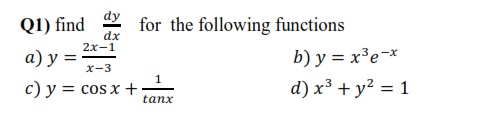dy dx 2x-1 Q1) find for the following functions a) y = b) y = x3e-* c) y = cos x + d) x3 + y2 = 1 x-3 1 tanx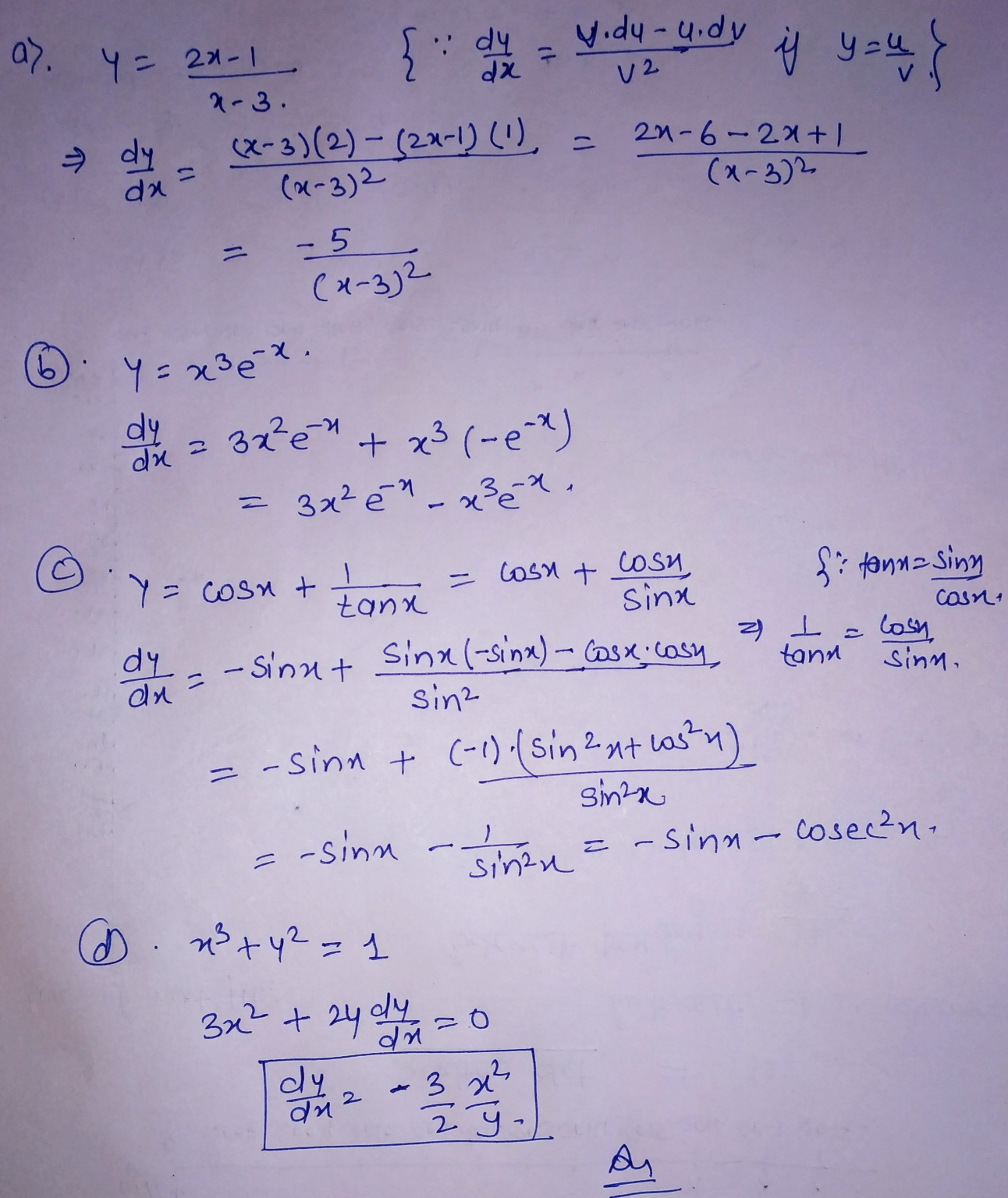##### Add Answer of: dy dx 2x-1 Q1) find for the following functions a) y = b) y = x3e-*...
Similar Homework Help Questions
• ### )Find dy/dx when y= Ln (sinh 2x) my answer >> 2coth 2x

1.)Find dy/dx when y= Ln (sinh 2x) my answer >> 2coth 2x. 2.)Find dy/dx when sinh 3y=cos 2x A.-2 sin 2x B.-2 sin 2x / sinh 3y C.-2/3tan (2x/3y) D.-2sin2x / 3 cosh 3yz...>> my answer. 2).Find the derivative of y=cos(x^2) with respect to x. A.-sin (2x) B.-2x sin (x^2)<<<< my answer. C.-sin (2x) cos (x^2) D.-2x sin (x^2) cos (x^2) 3).Find the derivative of y=sin^2(4x) cos (3x) with respect to x. A. 8 sin (4x) cos (3x) - 3...

• ### find the integral secxtanxdx and if dy/dx= 2x/y^2 and y(o) = 3 then what is y(1) equal to and differentiate 2pi + 3secx secxtanxdx = Sin(x)/Cos^2(x)dx The integral is 1/Cos(x) + c dy/dx= 2x/y^2 and y(o) = 3 then what is y(1) equal to

find the integral secxtanxdx and if dy/dx= 2x/y^2 and y(o) = 3 then what is y(1) equal to and differentiate 2pi + 3secxsecxtanxdx = Sin(x)/Cos^2(x)dx The integral is 1/Cos(x) + c dy/dx= 2x/y^2 and y(o) = 3 then what is y(1) equal to y^2 dy = 2x dx ---> 1/3 y^3 = x^2 + c y(0) = 3 ---> c = 9

• ### Q1: Solve the ODE: f) Vyy' + y3/2-1. y(1) = 0. g) (2x +y)dx(2x+y-1)dy 0. i) dx=xy2e": y(2)=0. j) ...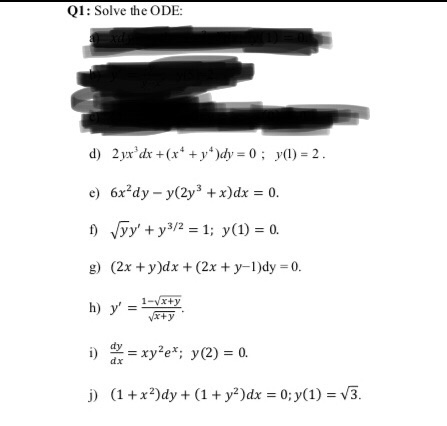Q1: Solve the ODE: f) Vyy' + y3/2-1. y(1) = 0. g) (2x +y)dx(2x+y-1)dy 0. i) dx=xy2e": y(2)=0. j) (1 + x*)dy + (1 + y*)dx = 0; y(1) = V3. Q1: Solve the ODE: f) Vyy' + y3/2-1. y(1) = 0. g) (2x +y)dx(2x+y-1)dy 0. i) dx=xy2e": y(2)=0. j) (1 + x*)dy + (1 + y*)dx = 0; y(1) = V3.

• ### Find dy/dx Differentiation

Find dy/dx evaluated at the given values.y2-x3=1 at x=2, y=3

• ### Which one is the solution to this equation (1 + y2 sin 2x)dx – 2y(cos x){dy...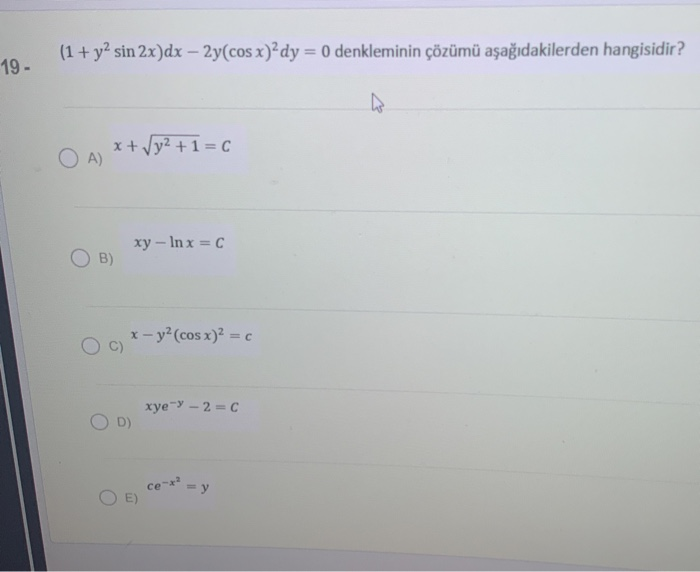Which one is the solution to this equation (1 + y2 sin 2x)dx – 2y(cos x){dy = 0 denkleminin çözümü aşağıdakilerden hangisidir? 19- x + √y²+1=c O A) xy - Inx=0 B) x-y(cos x)2 = C xye-y - 2 = 0 D) ce-x = y E

• ### 1a) Find dy/dx x = te', y = t + sin t b) Find dy/dx and...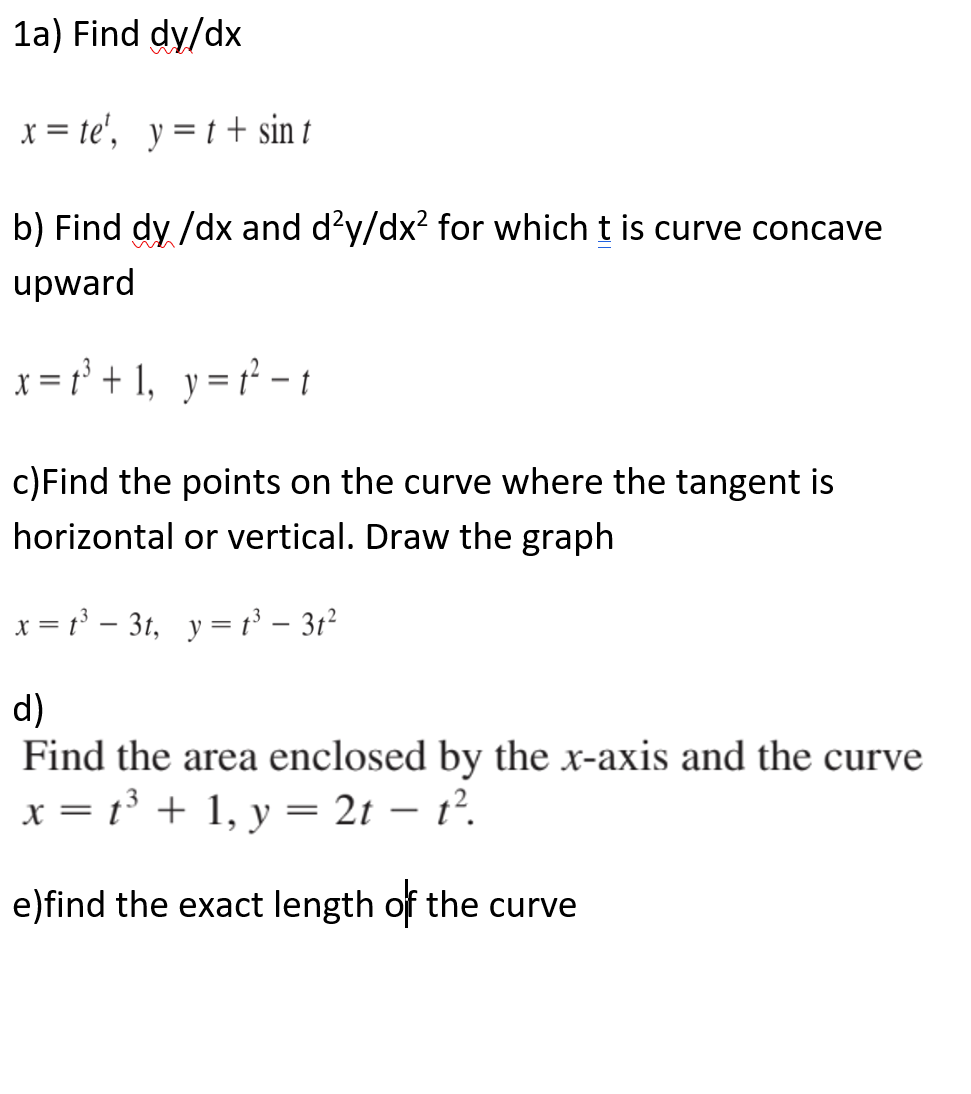1a) Find dy/dx x = te', y = t + sin t b) Find dy/dx and d’y/dx2 for which t is curve concave upward x = x3 + 1, y = t - c)Find the points on the curve where the tangent is horizontal or vertical. Draw the graph x = 13 – 3t, y = t3 – 312 d) Find the area enclosed by the x-axis and the curve x = t3 + 1, y = 2t – t?....

• ### Find the general solution or particular solution of each the following DE's 1) (y-y2 tanx)dx...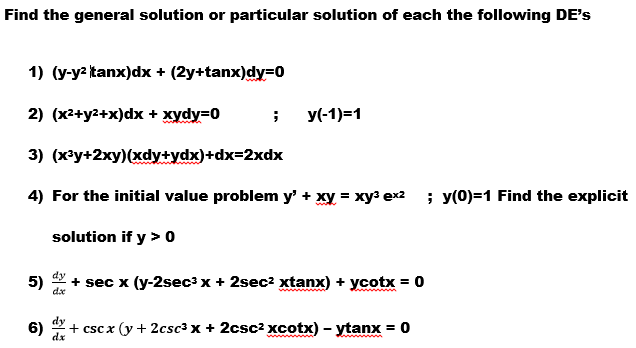Find the general solution or particular solution of each the following DE's 1) (y-y2 tanx)dx + (2y+tanx)dy=0 2) (x2+y2+x)dx + xydy-0 i y(-1)-1 4) For the initial value problem y' + xy - xy? ex2 ; y(0)-1 Find the explicit solution if y>0 dy dae dy

• ### Find dy/dx if y = (x^3)(e^2x)Find dy/dx if y = ln(x^2 + 5x)

Find dy/dx if y = (x^3)(e^2x)Find dy/dx if y = ln(x^2 + 5x)

• ### Problem 3: Find a solution to the IVP dy dy + dc2 + y = 0,...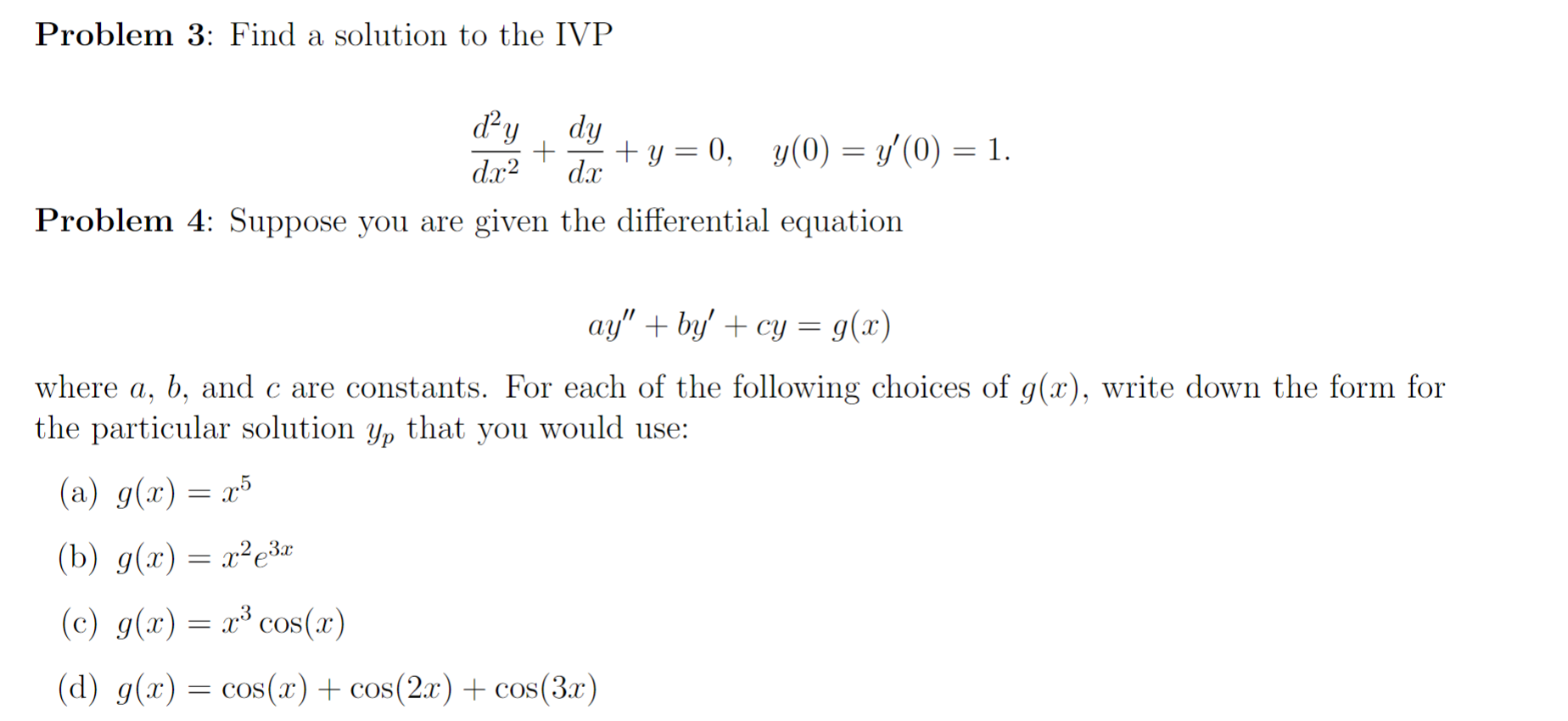Problem 3: Find a solution to the IVP dy dy + dc2 + y = 0, y(0) = y'(0) = 1. dx Problem 4: Suppose you are given the differential equation ay" +by' + cy = 9(2) where a, b, and c are constants. For each of the following choices of g(x), write down the form for the particular solution Yp that you would use: (a) g(x) = 205 (b) g(x) = x²e32 (c) g(x) = xº cos(x) (d) g(x)...

• ### 1. Let Q1 = y(7), where y solves dy dx + 8x 2 = 5x, y(6)...

1. Let Q1 = y(7), where y solves dy dx + 8x 2 = 5x, y(6) = 4. Let Q = ln(3 + |Q1|). Then T = 5 sin2 (100Q) satisfies:— (A) 0 ≤ T < 1. — (B) 1 ≤ T < 2. — (C) 2 ≤ T < 3. — (D) 3 ≤ T < 4. — (E) 4 ≤ T ≤ 5. 2. Let Q1 = y(1), where y solves dy dx + 1.7y = 5e 1.2x...

Free Homework App